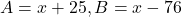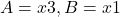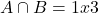## Solve (x + 2 < 5) ∩ (x - 7 > -6). A. {all real numbers} B. {x | 1 < x < 3} C. {x | x < 3 or x > 1}

Question

Solve (x + 2 < 5) ∩ (x – 7 > -6).

A. {all real numbers}
B. {x | 1 < x < 3}
C. {x | x < 3 or x > 1}

in progress 0
6 months 2021-07-21T13:02:44+00:00 2 Answers 20 views 0

Assuming b

Step-by-step explanation:

the person above said it was b so

2. Let.

Simplifying both you get.

The set conjunction is.